Technical
Technical
Technical

# The simplest model debiasing approach

June 6, 2022

By

Samuel Cohen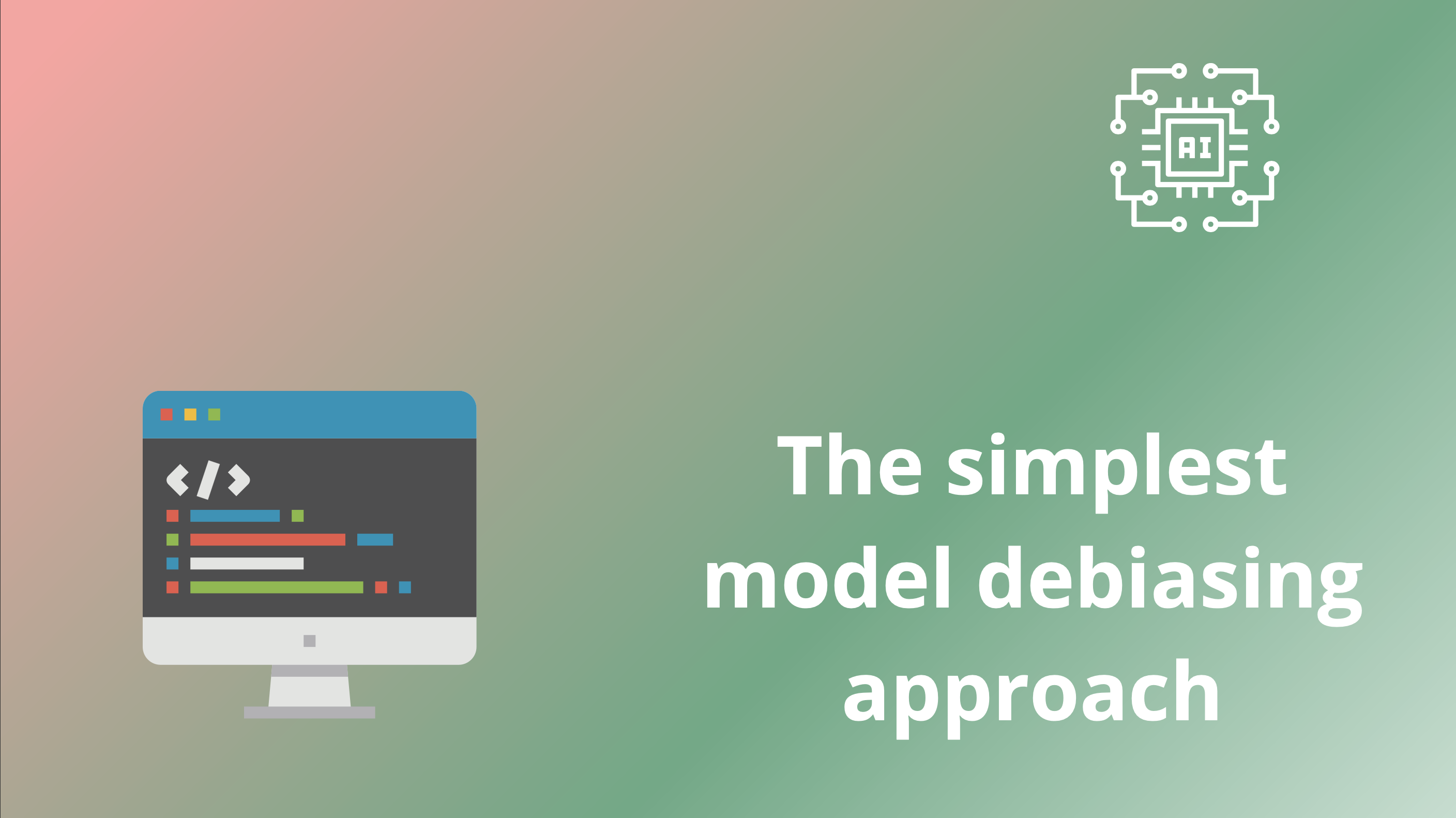Machine learning is used in most industries to automate decision processes, e.g., in banking, insurance and HR. Historical data is used to train models, and biases inherent to this data are replicated in the behaviour of the trained models.  In this blog post, we look at one of the simplest and intuitive model-centered methods for making the predictions of AI models fairer.

# The data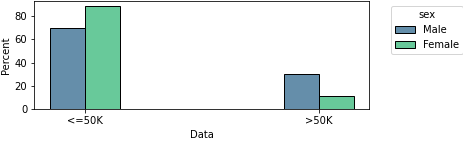Figure 1: Proportion of low- and high-income males and females in the US census dataset

We are interested in an income prediction task based on real US census data. We can see in Figure 1 that there is a gap of 19% in the proportion of high-income males and female.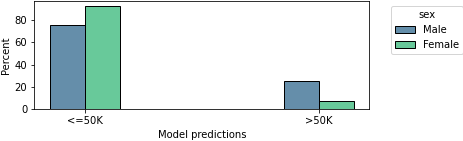Figure 2: Proportion of low- and high-income males and females as predicted by an ML model

When we train a ML model (logistic regression) on this data, and allow it to make decisions on a hold-out dataset, it allocates 18% more high-income predictions to males than females, replicating the unbalanced patterns from the training data.

Our aim is to train a model that will provide balanced high/low-income predictions, while preserving the accuracy (the proportion of correctly classified individuals) as much as possible.

# A simple debiasing solution

Most machine learning models not only provide binary high/low-income predictions, but also assign probabilities for each option. Typically, we set the threshold for predicting high- or low-income at the 50% level, meaning that if your probability of being high-income is estimated to be over 50%, then we assign a high-income prediction.

In order to force the model to allocate balanced high- and low-income predictions, we can use separate thresholds for males and females. In order to find these thresholds, we first look at the overall number of high-income individuals in the full population -- it is of 24%. We hence need to find a separate probability threshold for males and females that will lead to 24% of high-income (predicted) individuals in each group.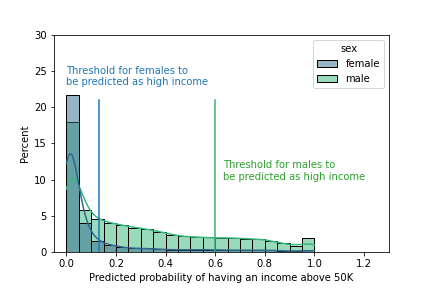Figure 4: the following thresholds allow both subgroups to have 24% of their members predicted as high-income

First, we begin by training a logistic regression on the training dataset. Second, we use this model to allocate probabilities of being high-income to each individual in the training data.  We then find the probability thresholds for males and females such that 24% in each group will be predicted a high-income (see Figure 4).

As can be seen in the figure, females with a 13% probability of having a high-income will be predicted high-income, and males with a 60% probability of having a high-income will be predicted a high-income.

We observe in Figure 5 that after this decision post-processing, we provide as many high-income predictions to each group, and the overall accuracy decreased of 2% only.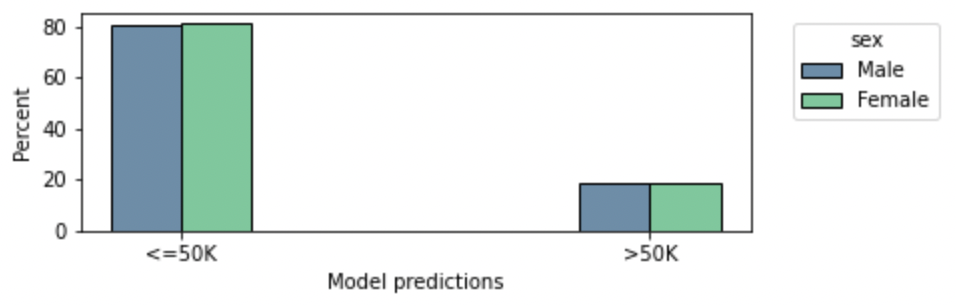Figure 5: By leveraging threshold-corrected income predictions, the model predicts the same proportion of low/high income individuals in each group while losing only 2% accuracy.

Get the code to run this experiment by yourself !